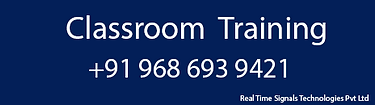# Matlab Training course

Prerequisites: Basic Computer and programming language

Course Details

Duration: 30 hours

Effort: hours per week

Price With GST: 17700/-

Subject:

Level: Beginner to Moderate

## Section 1: An Introduction to Matlab Software

• What is Matlab ?

• Matlab User Interface

• Saving Workspace Results in Matlab

• CLC, Home and Help Commands

• Running Scripts and Home Command

• Script File Extension

Section 2: Chapter 2- Math in Matlab

• Basic Arithmatic

• Math

• Variables in Matlab

• Variables

• Order of Operations in Matlab

• order of precedence in Matlab Operations

• Exponent & pi - Important friends

• pi & exponential

• Two sample Programs

• Symbolic Toolbox -1

• Symbolic Toolbox - 2

• Symbolic Toolbox - 3

Section 3: Variables Manipulation in Matlab

• Introduction to Variables

• Manipulating Variables

• Presenting results in different Formats

• Symbolic Variables

• Symbolic Calculations

• Symbolic Calculations

Section 4: Trigonometric Functions in Matlab

• Essential Functions

• Square Root

• Introduction to Trigonometry in Matlab

• Trigonometric Functions

• Hyperbolic Functions

• Exponential, Logarithm & Ln Functions

• Exponential

Section 5: Complex Numbers

• Introduction to Complex Numbers in Matlab

• Complex

• Complex Functions

• Complex 2

• Symbolic Toolbox and Complex Functions

• Complex 3

• Symbolic Calculation and Complex numbers

Section 6: Vectors

• Introduction to Vectors in Matlab

• Vectors Creation and Extraction

• Changing a Vector in Matlab

• Vector Calculations

• Dot & Cross Products and their difference

• Statistics on Vectors

• Vector Extraction & Manipulation

• Creating New Vectors

• Element by Element operation on vectors

• Mathematical Calculations & Vectors

• Random Vectors

• Statistical Analysis & Vectors

Section 7: Matrices

• Introduction to Matrices

• Data Extraction in a Matrix

• Matrix Multiplication

• Element & Element Multiplication in Matrices

• Max, Min, Number of Elements in a Matrix

• Matrix Augmentation

• Matrix & Important Functions

• Special Matrices

• Transpose & Diagonal Matrices

• Solving Equations using Matrices

• Trace, Inverse and More

• Symbolic Calculations and Matrices

Section 8: Introduction to Calculus, Engineering and Functions in MATLAB

• Defining New Functions

• Differentiation 1

• Symbolic Differentiation

• Integration

• Limit

• Partial Derivitives

Section 9: Graphs & Plots in Matlab

• Plotting 1

• Plotting 2

• Plotting 3

• Plotting 4

• Matlab Plots - The easy way

Section 10: Loops, Conditions and Programming in Matlab

• Logical and Relational Operations 1

• Logical & Relational Operations 2

• Introduction to Else in Matlab

• Introduction to Else-If -1

• Introduction to Else-If -2 - With Examples

• Introduction to " While" -with Examples

• For Loops

Section 11: Matlab Projects - Solved Examples with Code

• Project 1- Creating Functions in Programs

• Project 2- Creating a Function to evaluate a Polynomial for any number

• Project 3 - Automate finding the area of a triangle for any base and height

• Project 4: More on Loops-Source Code Included

Training MATLAB Simulink DSP communication system image processing video training, matlab training and placement in bangalore, matlab simulink training institutes in bangalore, matlab training institute in bangalore, matlab course fees in bangalore, , Matlab training in bangalore